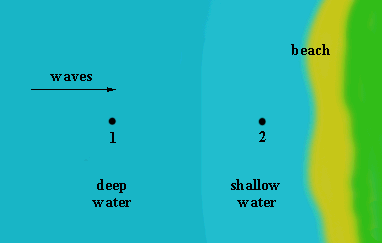# Constancy of Wave Period

As surface water waves travel from deep to shallow regions most of their characteristics change. For example, their celerity (speed) decreases, their wave length decreases, their shapes change, and their energies become re-distributed. There is one property, however, that remains unaltered: the wave period. If you observe the passing of wave crests at some point over deep water, and later observe the same crests as they pass over shallow water, you will see that the time between consecutive crests is exactly the same. Here is a proof.As they arrive at point 1 in deep water, their period is T1. If you count the number of crests that pass point 1 during some interval 'dt', you should get the number

`n1 = dt/T1                     Equation 1`

Suppose now that the wave period changes to the value T2 as the waves approach position 2 in shallower water. The number of crests passing point 2 in time dt is therefore

`n2 = dt/T2                     Equation 2`

The number of waves that have accumulated within the region between points 1 and 2 is the difference between the number entering the region and the number leaving the region, i.e.

`# of waves accumulated = n1 - n2 = dt*{1/T1 - 1/T2}     Equation 3`

Since the time interval dt is completely arbitrary, let it approach infinity. This makes the number of waves accumulated approach either plus infinity (for T1 < T2) or minus infinity (for T2 < T1). Neither result is physically acceptable. Hence, our original assumption that T1 and T2 are different must be invalid. This is a proof by contradiction.

Conclusion: the wave period is independent of the water depth